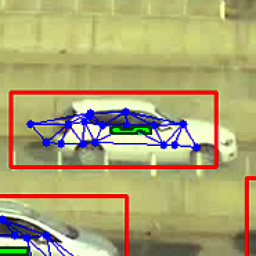# Niko's Project Corner

## Simulating gravitational field near a torus

 Description Fitting a 2D model on data sampled from 3D space Languages Matlab Tags Ap­plied math­emat­ics Duration Spring 2016 Modified 19th April 2016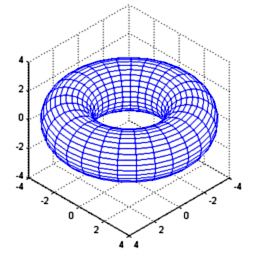There are many games with a strong em­pha­sis on grav­ity, and at times even multi-body tra­jec­tory sim­ula­tions. How­ever they all seem to be based on spher­ical ge­om­etry (as plan­ets are shaped by grav­ity), but other shapes should cre­ate in­ter­est­ing tra­jec­to­ries. As torus has ro­ta­tional sym­me­try its grav­ity field can be mod­elled on a 2D cross-sec­tion. In this pro­ject torus' field is es­ti­mated in 3D, pro­jected to 2D and in­ter­po­la­tion func­tions are fit­ted. The space- and time-ef­fi­cient model could be used in a game to do physics sim­ula­tion in real time.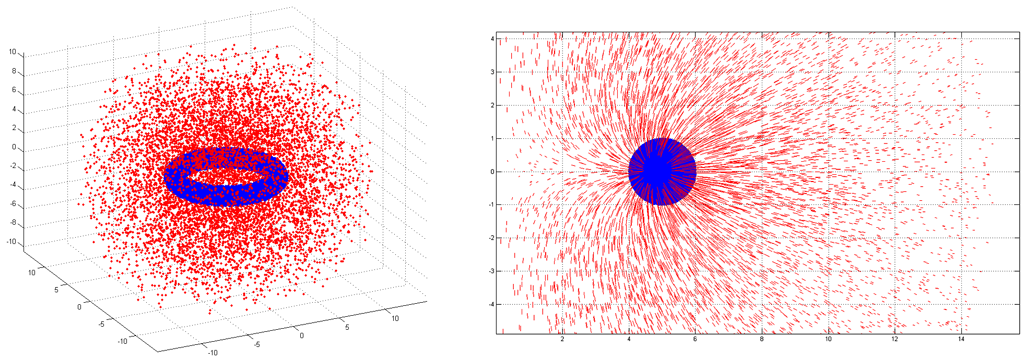Figure 1: Sam­pling ran­dom points within the torus and the space out­side it (on left). Due to ro­ta­tional sym­me­try they can be pro­jected and rep­resed on a 2D cross-sec­tion (on right).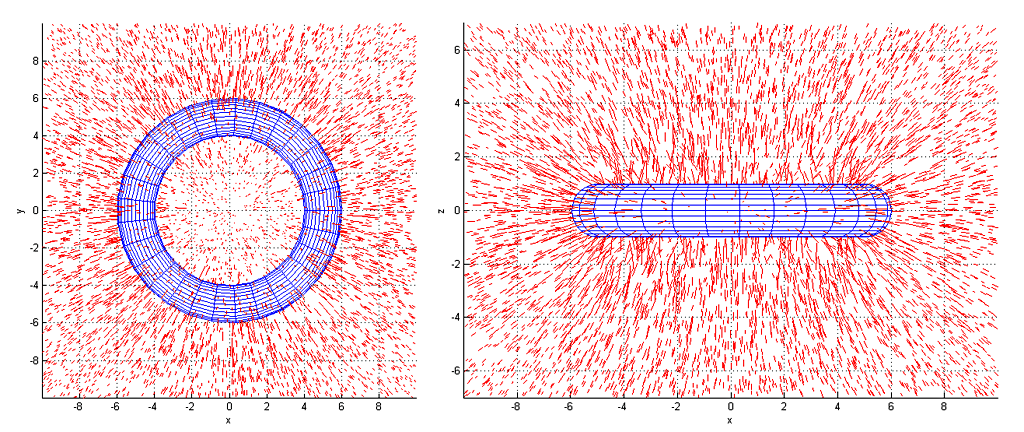Figure 2: Vi­su­al­iza­tion of grav­ita­tional force field around the torus, shown in XY and XZ planes.

In­stead of us­ing nu­mer­ical in­te­gra­tion this sim­pler method was used as I wasn't sure I wouldn't in­tro­duce un­in­tended bi­ases when defin­ing the in­te­gral over the vol­ume of a torus. The pro­cess starts from gen­er­at­ing ran­dom points within the torus (shown as blue in Fig­ure 1) and out­side it (shown in red). For each point out­side the torus the grav­ita­tional field's force vec­tor is es­ti­mated. These are then pro­jected to a 2D cross-sec­tion, as shown on the right side of Fig­ure 1. 3D plots of fields are shown in Fig­ure 2. It can be seen to de­cay to­wards zero (as ex­pected) at greater dis­tances, but in­ter­est­ingly field goes to zero also at the origin due to the sym­me­try.

Af­ter 3D co­or­di­nates have been ro­tated to the 2D cross-sec­tion plane, co­or­di­nates are trans­formed log-po­lar co­or­di­nates. These are shown in Fig­ure 3 (forces along x-axis) and Fig­ure 4 (forces along y-axis). Their carte­sian-co­or­di­nate coun­ter-parts are shown in Fig­ure 5. Shape of these graphs is a bit more ap­par­ent if they were seen in a ro­tat­ing 3D an­ima­tion. Red dots are cor­rect val­ues and blue val­ues are fore­casted by the fit­ted in­ter­po­la­tion model. There is some resid­ual er­ror but it shouldn't be ap­par­ent in a game-like sim­ula­tion.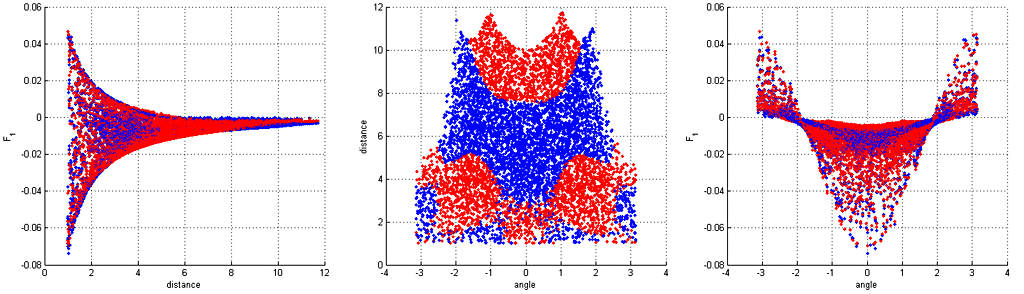Figure 3: Grav­ita­tional field's force along the x-axis (in cross sec­tion co­or­di­nates).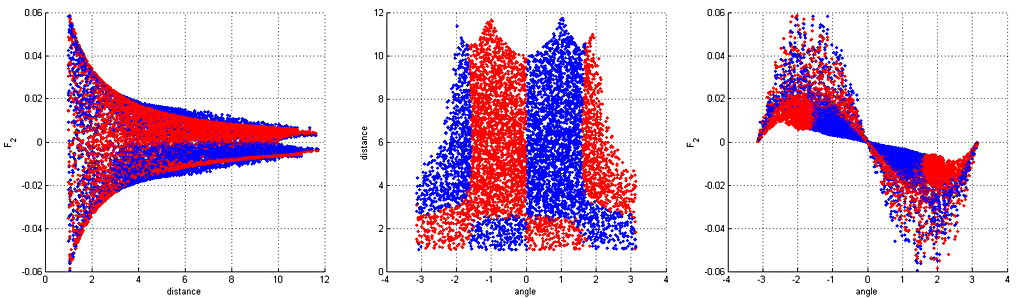Figure 4: Grav­ita­tional field's force along the y-axis (in cross sec­tion co­or­di­nates).

The model for the force along axises have the fol­low­ing forms:

Fx(α, d; p) → (p1cos(α)+ p2cos(2α)+p3) · exp(p4 · dp6) + p5 d + p7(1)
Fy(α, d; p) → (p1sin(α) + p2sin(3α)) · exp(p3 · dp4 + p5cos(α) + p6cos(2α))(2)

Val­ues for p at these two func­tions have the fol­low­ing val­ues:

• Fx: [-2.1924 0.1201 -0.3083 -3.6119 5.4967e-04 0.2802 -0.0073]
• Fy: [-15.0000 -1.4790 0.1457 -5.5883 -0.2798 -0.1553]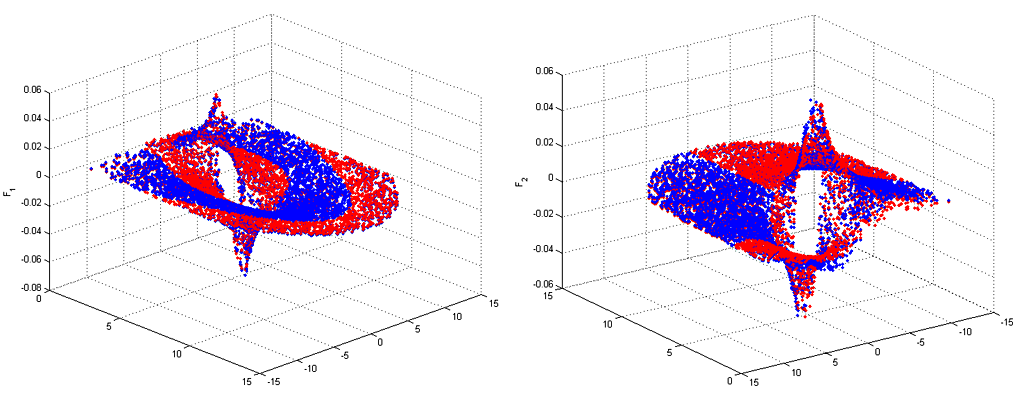Figure 5: Force vec­tors' x- and y-axis com­po­nents plot­ted in carte­sian co­or­di­nates.

This ge­om­etry cre­ates a field which have some in­ter­est­ing prop­er­ties, when com­pared to the "stan­dard" in­verse square law (1/r2) of a spherical planet or a star. Four examples of these are shown in figures 6 - 10. There seems to exist various classes of non-closed trajectories as one could say torus has two degrees of "freedom" instead of just the one on spheres. I was happy to discover that there exists orbits which go through the hole in the middle (figures 9 and 10).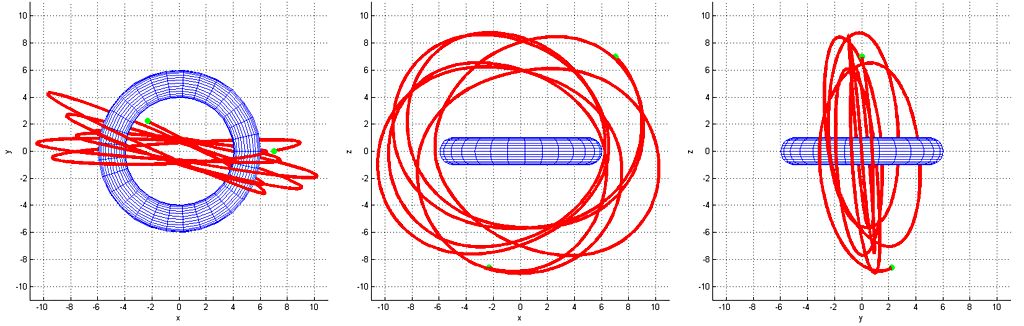Figure 6: A quite cir­cu­lar tra­jec­tory on the XZ-plane.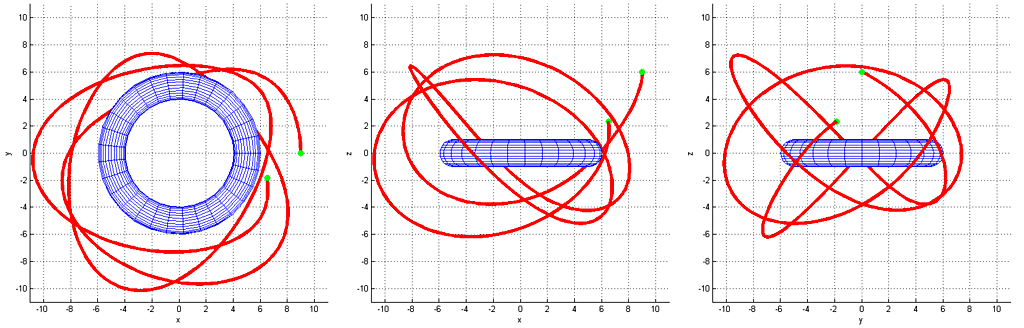Figure 7: A mod­er­ately chaotic tra­jec­tory, go­ing back-and-forth on all di­men­sions.

Tra­jec­to­ries were es­ti­mated by run­ning the dif­fer­en­tial equa­tion through the stan­dard mid­point method with a suit­ably small time-step. Most tra­jec­to­ries seem to be fairly chaotic and un­sta­ble, so sim­ulat­ing ad­di­tional bod­ies such as moons might have non-in­ter­est­ing re­sults. It would be in­ter­est­ing to sim­ulate an at­mo­sphere con­sist­ing of ideal gas but I have no prior ex­pe­ri­ence on that even on a spher­ical body. It would also be worth study­ing game-me­chan­ics on the FPS genre, and why not also for a plat­former?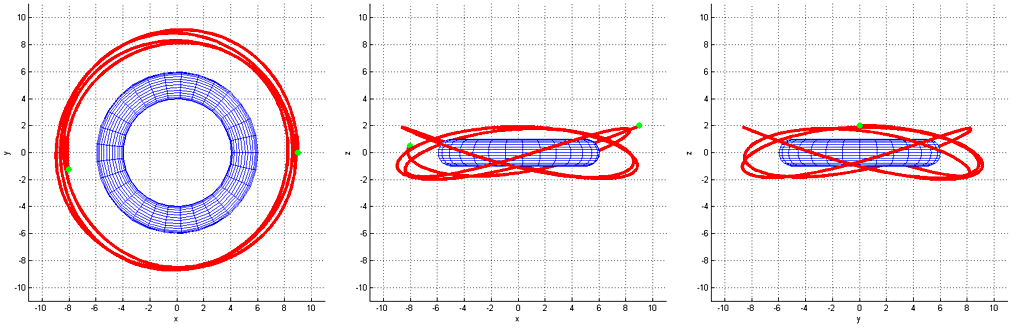Figure 8: A cir­cu­lar tra­jec­tory on the XY-plane.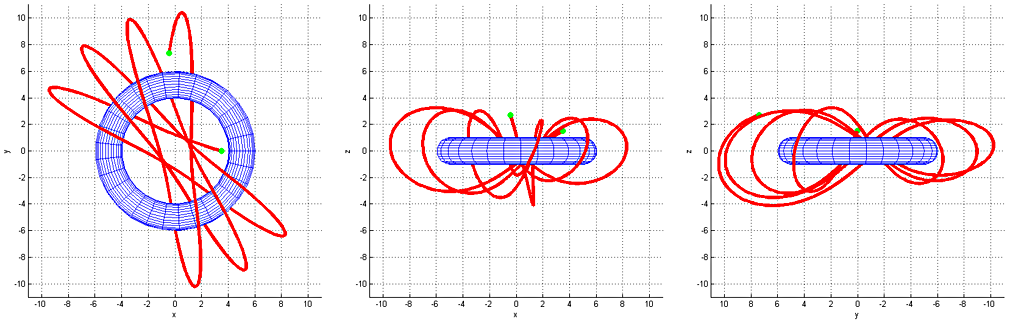Figure 9: An in­ter­est­ing tra­jec­tory which goes through the torus' hole at the mid­dle.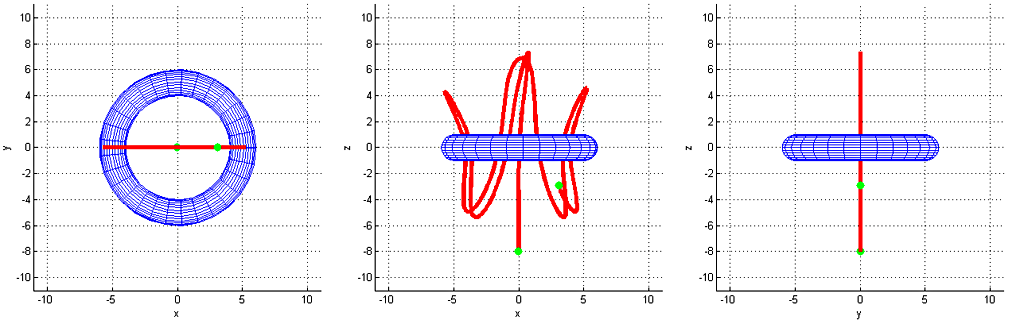Figure 10: An os­cil­lat­ing path on the XZ-plane.

#### Related blog posts:

 Satellite crash course, 2021 Jun (Matching: Applied mathematics)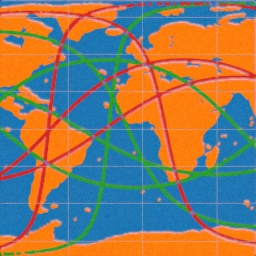Approximating planets' orbits in closed-form, 2014 Oct (Matching: Applied mathematics, Matlab)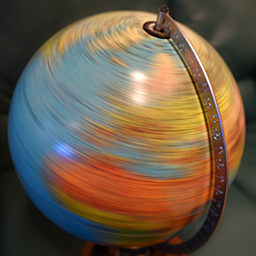Automatic map stitching, 2014 Sep (Matching: Matlab)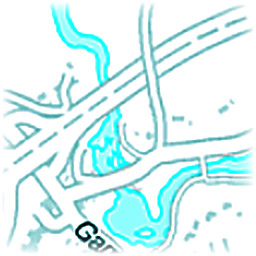Image distortion estimation and compensation, 2014 Aug (Matching: Matlab)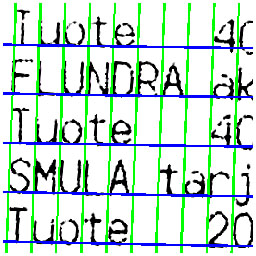Real-time car tracking and counting, 2014 Jun (Matching: Matlab)